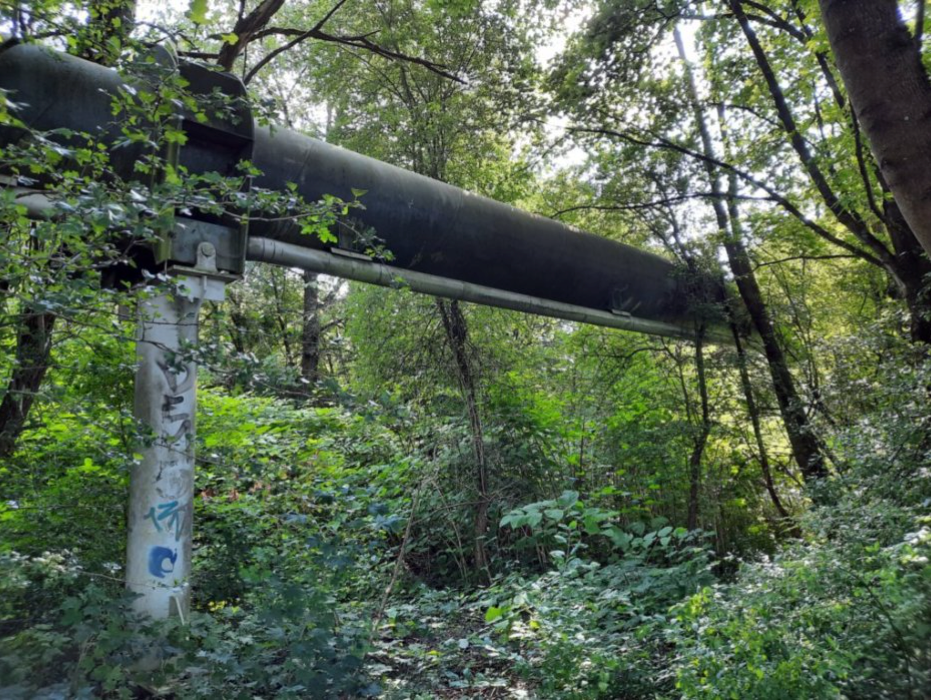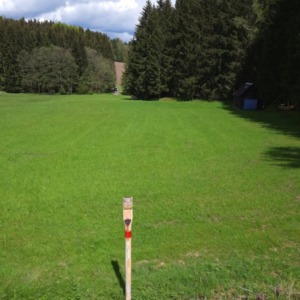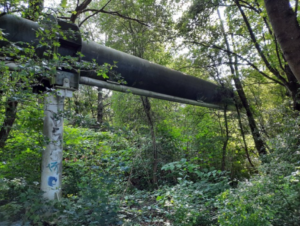# Modeling, Robust Design and Control of Gas Networks# Doctoral School of Information and Biomedical Technologies Polish Academy of Sciences: Modeling, Robust Design and Control of Gas Networks

• Jan Sokolowski, Martin Gugat
• Supported by –
• Duration: 2022 –

Simulation and Optimization on Finite Graphs is considered for the example of Gas Networks.

We are interested in two models defined on the graph. The first model for steady state solutions. The second model for evolution problems. The steady state solutions are considered for the geometrical optimization and robust design
of the structure. The location of nodes and the length of vertices are selected at this stage. The more complex model for evolution problems is considered for control and management of the network. At this stage the location
of compressors is selected on some vertices of the network.

The model is a distributed parameter system with solutions depending on the space variable in the static case. In the dynamic case the evolution in time is considered. The possible connection between two models is the objective function
that pull the system towards a static state, which results in the turnpike phenomenon.

Place of research: Warsaw (Poland) and Erlangen (Germany)

# Scope/Goal

The geometry of finite graphs is optimized for a given task characterized by its cost. The task is a control problem or an optimization problem in the static case. Modeling of gas flow and coupling conditions for flow through vertices is considered over a given network. Gas models are considered for spatially one-dimensional flow governed by hyperbolic balance laws. The classical solutions of the model are considered as well as weak solutions. The networks are described by finite graphs. The stationary states of the model of gas networks are used for the design of the graph.

The tools of shape optimization are applied to the shape and topology optimization of finite graphs. The model considered is well posed for a fixed geometry of the graph. The optimal value of the cost for the optimization problem for the gas network depends on the geometry of the graph. The design of the network means the selection of an admissible geometry of the graph which minimizes the given shape functional. If the gas network is considered with random demands,
the stochastic optimization methodology can be applied.